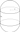# Capsule Volume and Area Calculator

Height

Select Unit### How To Calculate Capsule Volume?

Capsule Volume =π * radius2 * ( 4/3 * radius + height) .
ex : Rectangle have radius = 15, height = 20 .
Volume = π * 152 * ( 4/3 * 15 + 20) = 28274.33 m3
.
π = 3.14159265359
The capsule is a basic three-dimensional geometric shape consisting of a cylinder with hemispherical ends.

### How To Calculate Capsule area?

The surface area formula =2 * π * radius * ( 2 * radius + height) .

### How To Calculate Capsule lenght ?

Capsule lenght = 2 * radius + height .